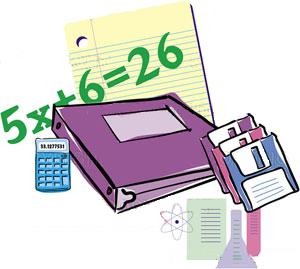# Variables and Algebra

##BIG IDEAS:

(taken from “Big Ideas by Dr. Small”):

1. Algebra is a way to represent and explain mathematical relationships and to describe and analyze change.
2. Using variables is a way to efficiently and generally describe relationships that can also be described using words.

## STUDENT LEARNING GOALS:

GOAL #1: I can write algebraic expressions and equations from words.

GOAL #2: I can evaluate algebraic expressions using substitution.

GOAL #3: I can solve one- and two-step algebraic equations.

## CURRICULUM EXPECTATIONS:

• Model real-life linear relationships graphically and algebraically, and solve simple algebraic equations using a variety of strategies, including inspection and guess and check;
• Translate phrases describing simple mathematical relationships into algebraic expressions (e.g., one more than three times a number can be written algebraically as 3x + 1), using concrete materials (e.g., algebra tiles, pattern blocks, counters);
• Evaluate algebraic expressions by substituting natural numbers for the variables;
• Solve linear equations of the form ax = c or c = ax and ax + b = c or variations such as b + ax = c and c = bx + a (where a, b, and c are natural numbers) by modelling with concrete materials, by inspection, or by guess and check, with and without the aid of a calculator (e.g., “I solved x + 7 = 15 by using guess and check. First I tried 6 for x. Since I knew that 6 plus 7 equals 13 and 13, is less than 15, then I knew that x must be greater than 6.”).
• Model real-life relationships involving constant rates (e.g., speed, heart rate, billing rate), using algebraic equations with variables to represent the changing quantities in the relationship (e.g., the equation p = 4t represents the relationship between the total number of people that can be seated (p) and the number of tables (t), given that each table can seat 4 people [4 people per table is the constant rate]).